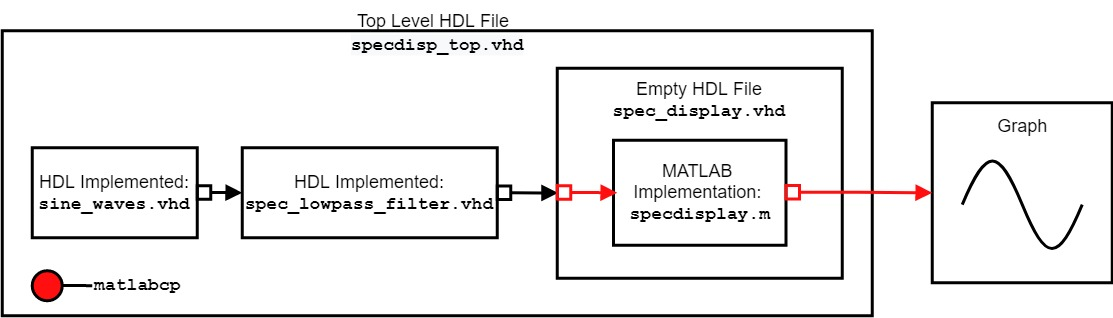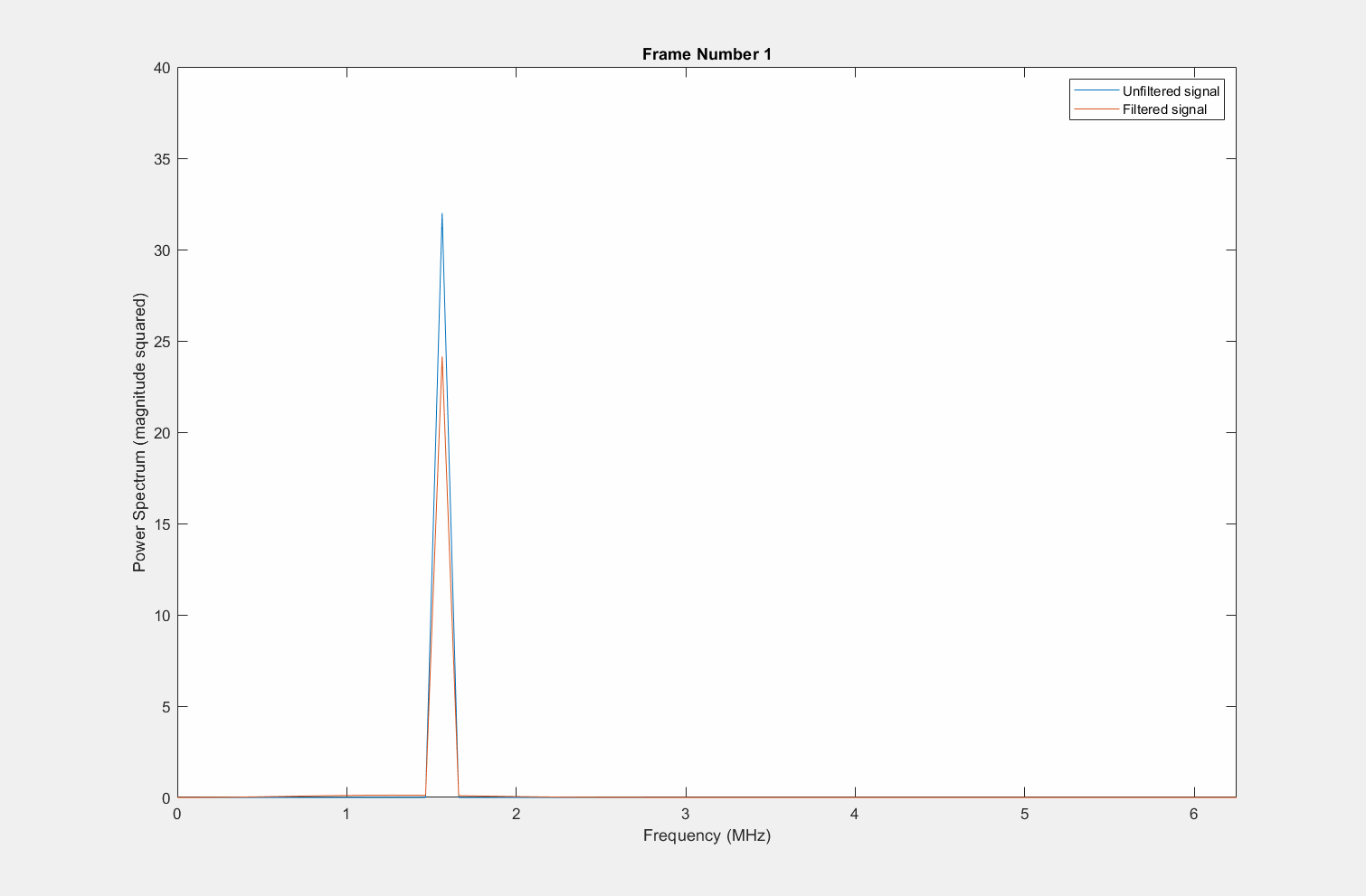# Implement Spectrum Display Component in MATLAB

This example shows how to run MATLAB® with Mentor Graphics® ModelSim® and Questa® or Cadence® Xcelium™ to visualize the output of a larger HDL project, by using the HDL Verifier™ function `matlabcp`. Visualizing and analyzing the output of the HDL module using the host of scopes, plots, and viewers in MATLAB accelerate the development of the HDL module and its verification. In this example, you use the `matlabcp` function to bind the HDL module with its output. You visualize the HDL module output using the `plot` function in MATLAB.

In this example, you use `matlabcp` to visualize the spectrum of a signal from a VHDL® project, which consists of a sine wave generator, a low-pass filter, and an empty HDL file representing the spectrum display component. `matlabcp` uses the empty HDL file to send the project output into a MATLAB function, which buffers the data into frames of 128 samples. Each time a frame is completed, the MATLAB function plots the spectrum of the unfiltered and filtered data in the frame. This use of `matlabcp` for reading the HDL data streamlines the transition between raw simulator data and visual plot, as MATLAB performs the data processing and graphic creation in parallel with the HDL simulator. This diagram shows an overview of the cosimulation system.This example considers the point of view of an HDL developer in mind, so the main emphasis of this example is on using the HDL simulator and command-line terminal to perform testing and using MATLAB to manipulate and visualize the data from the HDL simulation.

### Overview of Design and Script Files

This example uses two types of files: design files and script files.

Design Files

• The file `sine_waves.vhd` contains a frequency hopping sine wave implemented in VHDL.

• The file `spec_lowpass_filter.vhd` contains a low-pass filter.

• The file `spec_display.vhd` contains the empty component that `matlabcp` uses to feed the VHDL data into the MATLAB code.

• The file `specdisp_top.vhd` contains the top-level wiring between the signal source, low-pass filter, and empty component for `matlabcp`.

• The file `specdisplay.m` contains the MATLAB code that processes the inputs from the HDL simulator and plots their spectrum.

Script Files

• The file `qcommands_spec.tcl` contains the TCL commands that are sent into ModelSim/QuestaSim for cosimulation to occur.

• The file `tclcmds_spec_xc.m` contains the TCL commands that are sent into Xcelium for cosimulation to occur.

Additionally, this example requires ModelSim/QuestaSim or Xcelium and MATLAB to be on the system path, as you must be able to run these products from the terminal.

### Bind MATLAB Function with HDL Module

You can bind a MATLAB function with an HDL module using `matlabcp`. You need an empty HDL component with input and output ports declared. These files are necessary as `matlabcp` uses the empty HDL component as a shell for communication between MATLAB and the HDL simulator, while the MATLAB function provides the functionality of the component. Using `matlabcp` allows MATLAB to process the simulator data as the simulation progress, saving time needed to transform the original outputs into a visual format. For information on creating the necessary files for `matlabcp`, see Create a MATLAB Component Function.

### Run the Cosimulation

To start cosimulation, open MATLAB and run the HDL Link MATLAB server for communication between the HDL simulator and MATLAB. To complete these actions, use these commands based on the operating system.

• Windows: `matlab -nodesktop -r "hdldaemon"`

• Linux: `xterm -e "matlab -nodesktop -r "hdldaemon"" &`

This command opens MATLAB in a separate terminal, in headless mode, while the HDL Link MATLAB server starts. The terminal that is running MATLAB displays this message:

`"HDLDaemon shared memory server is running with 0 connections"`

Next, start the cosimulation. Enter the applicable command (based on the simulator and operating system) into MATLAB command window.

ModelSim/QuestaSim

• Windows and Linux:

```Launch ModelSim/QuestaSim and execute TCL commands in the simulator by entering this command.```
```vsim('tclstart','do qcommands_spec.tcl'); ```

Xcelium

• Linux:

1. Run the MATLAB script `tclcmds_spec_xc.m` by entering this command at the MATLAB command prompt.

```tclcmds_spec_xc; ```

2. Launch Xcelium in batch mode by entering this command at the MATLAB command prompt.

```nclaunch('tclstart', tclcmds); ```

This command starts the cosimulation. Over the course of the simulation, MATLAB captures, processes, and displays multiple frames. The plot shows a comparison between the power spectrum of the unfiltered signal and the filtered signal. Observe that as the frequency approaches higher values, the filtered signal diminishes and eventually becomes zero at a certain point. This behavior is typical for a low-pass filter.### Summary

This example shows how to use MATLAB to interpret and display results after the HDL simulation has finished processing. Even though the simulator can display the unmodified results of the project, applying transformations to the results for visualization can be difficult. In this example, the spectrum of the frequency hopping sine wave was desired, but to obtain this spectrum, a Fourier transform is required. This requirement significantly complicates the process of coding, simulating, and verifying the results.

Using MATLAB and `matlabcp` can significantly speed up this visualization, as MATLAB can read data from the simulation and utilize built-in functionality to apply the Fourier transform and display the results. By using MATLAB in the verification process, the complexity of visualizing the data is reduced, streamlining the process of converting simulation results into visualized data.